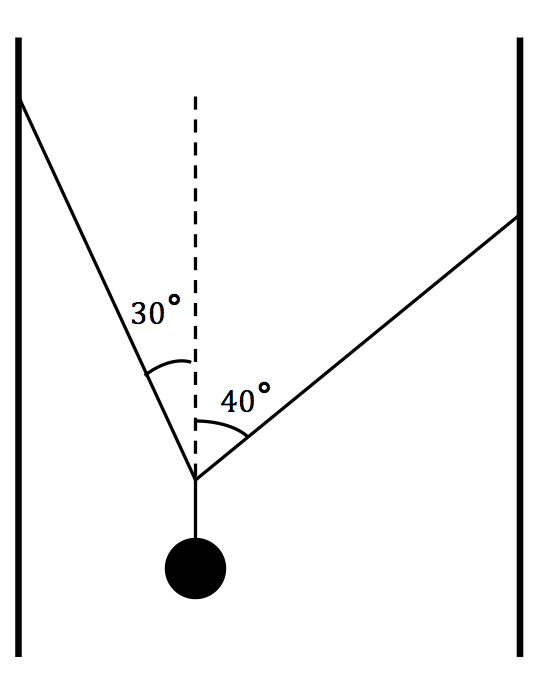# Particle Held In The Air By StringA particle of mass $1\text{ kg}$, is held in the air by two pieces of string attached to the wall. (As shown in the diagram above).

If the 2 strings have tension $T_1$ and $T_2$ respectively, what is $|T_1| + |T_2|$?

Assume $g=10 \text{ m/s}^2$.